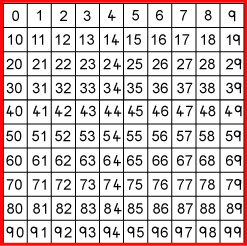#### You may also like### Consecutive Numbers

An investigation involving adding and subtracting sets of consecutive numbers. Lots to find out, lots to explore.### Roll These Dice

Roll two red dice and a green dice. Add the two numbers on the red dice and take away the number on the green. What are all the different possible answers?### Domino Square

Use the 'double-3 down' dominoes to make a square so that each side has eight dots.

# What Do You Need?

##### Age 7 to 11 Challenge Level:

Can you sort out the four clues that help and the four clues that do not help in finding the number I am thinking of?Four of the clues below are true but do nothing to help in finding the number.
Four of the clues are necessary for finding it.

Here are eight clues to use:
1. The number is greater than $9$.
2. The number is not a multiple of $10$.
3. The number is a multiple of $7$.
4. The number is odd.
5. The number is not a multiple of $11$.
6. The number is less than $200$.
7. Its ones digit is larger than its tens digit.
8. Its tens digit is odd.
What is the number?